🏆 Finlands bästa digitala läromedel 🏆

Läs mer om Finlands bästa digitala läromedel

# Högklassiga digitala matteböcker i en digital omgivning

Använd de färdiga prisbelönta matteböckerna, ändra på dem enligt eget behov, eller skapa helt nya!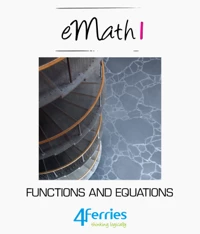## eMath 1 – Functions and equations

eMath1 is a repetition of middle school math, with some topics being treated more carefully than before. It gives an overview of the basic number domains and their operation, shows how solve first degree equations, how to work with percentages, roots and powers, and finally explains the important notion of a function.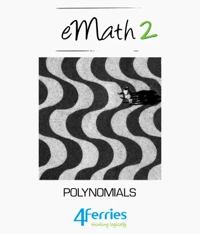## eMath 2 – Polynomials

This book presents first degree and higher degree polynomials. It shows how to solve equations involving polynomials, and how to factories polynomials. It also explains how to solve simultaneous equations as well as inequalities, and how to work with rational expressions.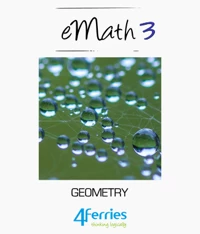## eMath 3 – Geometry

This book gives an overview of the basic concepts of plane geometry, and describes the theory of triangles, general polygons, and circles. It continues with an overview of solid geometry, with applications to cylinders, cones and spheres.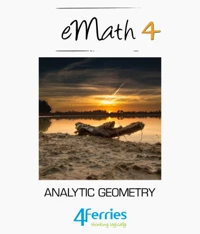## eMath 4 – Analytic geometry

This book introduces the basic notions of analytic geometry with the cartesian coordinate system. It shows how to describe lines as equations, and how to determine properties of lines, like intersection, orthogonality, distance from points and angles between lines. The book shows how to define and analyse properties of parabolas, circles and other conic sections.## eMath 5 – Vectors

The book gives an overview of vectors and vector spaces. It explains the basic notion of a vector, how to compute with vectors and how to describe them in a coordinate system. Three-dimensional vectors are also described, together with planes and lines in space.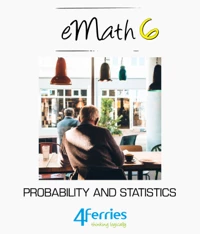## eMath 6 – Probability and Statistics

This book gives an overview of the basic notions of probability and statistics. The basic statistical measures are covered, as well as surveys and samples. The concept of probability is explained, together with the basics of combinatorics. The rules of probability are explained, to show how to compute with probabilities, and the notion of a random variable is explained.## eMath 7 – Derivatives

This book introduces the basic notions of calculus – continuity, limits, and derivatives. It shows how to compute the derivative of a function. It applies this to describe the behaviour of functions, and to find extreme values of functions. Additionally, it includes a chapter on how to work with rational expressions.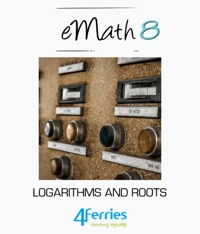## eMath 8 – Logarithms and Roots

This book describes how to work with exponents, logarithms and roots. It explains the notions of compound functions and inverse functions. It introduces exponential functions and logarithmic functions and shows how compute the derivative of these.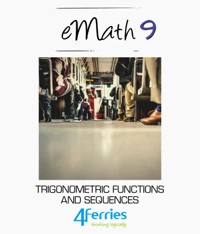## eMath 9 – Trigonometric Functions and Sequences

This book gives a more in-depth treatment of trigonometry. It covers the basic trigonometric functions, how to compute with these and how to solve equations with trigonometric functions. The book also covers sequences in general, particularly arithmetic and geometric sequences, as well as arithmetic and geometric sums.## eMath 10 – Integral Calculus

This book shows how to define integrals as the inverse of derivatives. It introduces the notion of a definite integral, and shows how to use this in area calculations. Definite integrals are then used to also calculate volumes of solids.